RS01 Solid sphere, 0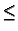rb, with G <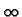(natural boundary) at r = 0, and G = 0 (Dirichlet) at r = b.

4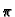GRS10(r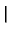r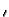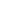) =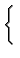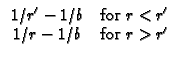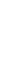RS02 Solid sphere, 0rb, with G <(natural boundary) at r = 0, and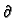G/r = 0 (Neumann) at r = b . Note that this geometry requires a pseudo GF, denoted H. The temperature solution found from a pseudo GF requires that the total volumetric heat flow is equal to the boundary heat flow, and the spatial average temperature in the body must be supplied as a known condition.

4GRS20(rr) =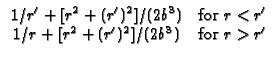RS03 Solid sphere, 0rb, with G <(natural boundary) at r = 0 and kG/r + h2G = 0 (convection) at r = b. Note B2 = h2b/k.

4GRS03(rr) =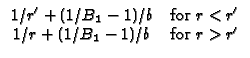Kevin D. Cole
2002-12-31